Back to the Table of Contents

# Triangle Congruences. Not!

#### Lesson Overview

Congruence between two triangles means six items, all three sides and all three angles, are congruent. Thus the statement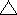ABC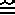DEF has a very precise meaning. It succinctly summarizes the six statements (assume lines atop these segments): ABDE, BCEF, ACDF,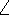AD,BE, andCF. It is very important to maintain the vertices in the proper order. Not doing so is a common mistake.

A typical proof using triangle congruence will use three steps to set up the three congruent triangle parts (several may be givens), a fourth step invoking a triangle congruence theorem, followed by a CPCF (Congruent Parts of Congruent Figures are congruent) invocation to relate additional congruent triangle parts. This kind of proof is very similar to those using transitivity in that regard and lend themselves nicely to the two column format. However, outside geometry most proofs are written in paragraph style. Our text advises against including givens to cut down on thoughtless ritual. (Thus they deduce conclusions instead of making statements.) However, as a visual learner, I tend to disagree with the authors on this one. It can often be a useful way to organize what you know, making it easier to fill in what you don't. Thus we will be flexible in format and advise students to try a variety of approaches until they find what suits them. In this chapter the two column proof reigns supreme, however.

### Triangle Congruences: SSS, SAS, AAS=SAA, and ASA

Consider using the characters S or A to fill three positions. There are 8=23 different possibilities: SSS, SSA, SAS, SAA, ASS, ASA, AAS, AAA which correspond with the binary equivalents of 0-7 (which are usually written with 0's and 1's). Consider further that S stands for side and A stands for angle. The essence is that for some combinations of three from the three sides and three angles in a triangle we can establish congruence between two triangles.

Below we will discuss the three triangle non-congruences of AAA, and SSA=ASS further. First we will discuss the four triangle congruences of SSS, SAS, SAA (which is the same as and is usually referred to as AAS), and ASA.

SSS is more formally known as the Side-Side-Side Triangle Congruence Theorem (or maybe Edge-Edge-Edge Triangle Congruence Theorem).

 If in two triangles the three sides are pairwise congruent, then the triangles are congruent.

As with much of our textbook, it proves this using transformations (reflections preserve distance and the Kite Symmetry Theorem). This is important because it differs from Euclid's faulty superposition development. It also differs from other modern rigorous developments which use SAS as a postulate (Hilbert, Birkhoff). Whichever route you take to develop YOUR geometry, you should also be able to convince yourself using only a compass and straight-edge that SSS always yields congruence. The two triangles might have opposite orientation, but they will still be congruent. As implied by the faulty development of Euclid on this score, the proof of these triangle congruence theorems is more involved than the proofs we expect you to be able to write. However, we do expect you to be able to follow the proofs given. The SsA Triangle Congruence Theorem is the longest in our text and does not appear in many texts, including Euclid's Elements.

We spoke earlier about the 3-4-5 triangle being a right triangle. Of course, not all 3-4-5 triangles are going to be congruent because someone might use 3 attometers, 3 miles, or even 3 light-years. However, because of the Pythagorean Theorem these are all right triangles. (Personally, I have reservations about both attometers and light-years due to quantization of space-time and general relativity.) This is a fundamental property that given the three sides of a triangle, you have fixed the angles. This relates as well to the fact that triangles are rigid. Rigidity is an important property in the functionality of objects like doors, rafters, and gates.

SAS is more formally known as the Side-Angle-Side Triangle Congruence Theorem. Be sure the angle you are using is BETWEEN the two sides you are using. If sides AB and BC are used, angle B is the included angle. Order is important and is implied by the order the letters are specified.

 If in 2 triangles 2 sides and the included angle are pairwise congruent, then the triangles are congruent.

AAS is more formally known as the Angle-Angle-Side Triangle Congruence Theorem. The side used here is opposite the first angle.

 If in 2 triangles 2 angles and a non-included side are pairwise congruent, then the triangles are congruent.

ASA is more formally known as the Angle-Side-Angle Triangle Congruence Theorem. The side used here is BETWEEN the two angles you are using. If angle A and angle B are used, side AB is the included side.

 If in 2 triangles 2 angles and included sides are pairwise congruent, then the triangles are congruent.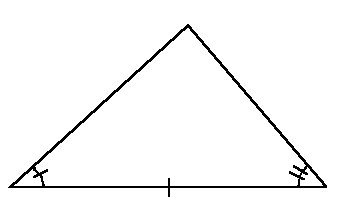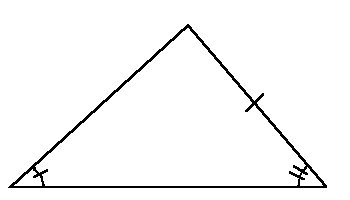ASA Triangle AAS Triangle

There is a fundamental difference between ASA and AAS which isn't readily apparent to the beginning geometry student. Consider the two triangles given above. Notice how the given side is between the two angles in the ASA triangle, whereas the given side is opposite one of the angles in the AAS triangle.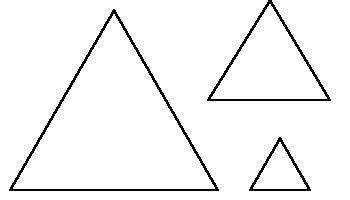### Triangle Non-congruences: AAA, and SSA=ASS

There is no Angle-Angle-Angle Triangle Congruence theorem. You should perhaps draw various equilateral triangles like those to the right to convince yourself of this. There is, however, an Angle-Angle-Angle Similarity Theorem. In fact, since if you know two angles, the third is fixed as 180°-the sum of their measure, it is known as the AA Similarity Theorem. This is discussed further in chapter 13.

 If in two triangles two angles are pairwise congruent, then the triangles are similar.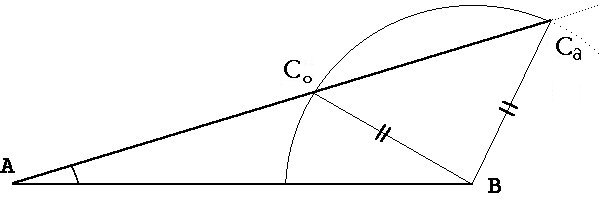There is NO SSA Triangle Triangle Congruence Theorem. (Although SSA and ASS are equivalent, please avoid the latter spelling, even though it fairly represents the situation should you invoke it.) This is what we refer to as the ambiguous case or SSA condition. You can think of condition as being like a disease. Please refer to the diagram above and note the following. Angle A is fixed (given). Side length AB is fixed (given). Side length BC is also fixed (given). However, there are two possibilities for C as indicated by where a circle centered at B intersects line AC. One is denoted Ca and the other Co. Ca results in an acute angle at C whereas Co results in an obtuse angle at C. (The opposite type of angle is formed at B, thus the triangle is always nonacute.) As long as BC is longer than the minimum distance between B and AC and shorter than AB, two triangles are possible. However, if BC is longer than AB only one triangle is possible (see SsA below). If BC is exactly equal to the minimum distance between B and AC, then only one triangle, a right triangle, is possible (see HL below). If BC is less than the minimum distance between B and AC, then no triangle is possible.

Just like there can be zero, one, or two solutions to a quadratic equation, there can be zero, one, or two triangles corresponding to a given SSA triple. This deeper connection is made more explicit by examining the quadratic nature of the Law of Cosines, a generalization of the Pythagorean Theorem.

 Law of Cosines: In triangle ABC with sides of length a, b, and c: c2 = a2 + b2 - 2abcosC.

Side a of length a=BC is oppositeA, side b of length b=AC is oppositeB, and side c of length c=AB is oppositeC. Notice how the Law of Cosines as stated is symmetric in a and b—they can be interchanged with the same result. Which angle/side is used is also arbitrary, so we could just as well have written it as: a2 = b2 + c2 - 2bccosA or b2 = a2 + c2 - 2accosB. Since the cos 90°=0, in a right triangle the Pythagorean Theorem results. Traditionally, in a right triangle, angle C is right and side c is then the hypotenuse. Thus the boxed statement of the law of cosines has additional merit.

### SSA Condition as HL or SsA

Our textbooks salvages two cases from the SSA condition as mentioned above. First, if BC is longer than AB it can intersect AC in only one point where angle C must be acute. This is commonly represented as the SsA Triangle Congruence Theorem where the longer side is opposite the given angle. Hence the upper and lower case S'es. In such a situation triangle congruence occurs.

 If in two triangles two sides and the angle opposite the longer of the two sides are pairwise congruent, then the triangles are congruent.

Second, if BC is exactly equal to the minimum distance between B and line AC, then angle C is a right angle. BC and AC are then legs of this right triangle and AB is the hypotenuse. Notice how if BC were slightly longer, two triangles would result and it if were slightly shorter no triangle is possible. This is commonly referred to as the HL Triangle Congruence Theorem.

 If in two right triangles the hypotenuse and a leg are pairwise congruent, then the triangles are congruent.

Other texts note that the SSA condition guarantees congruence if the congruent angles are nonacute (i.e. right or obtuse). With information at the end of this chapter (Law of Sines, etc.) it will be seen that these are equivalent. Note also how the HL Congurence Theorem is also a subset of SAS for right angles.

### Isosceles and Overlapping Triangles

Many tricks of the trade are involved in triangle congruence proofs. Vertical angles, corresponding angle, overlapping angles, etc. will often be used to find congruent angles. Rhombuses, rectangles, kites, and overlapping sides will often be used to find congruent sides.

With the AAS triangle congruence theorem we can now prove the converse of the Isosceles Triangle Base Angles Theorem or the converse of pons asinorum.

 If two angles of a triangle are congruent, then the sides opposite these angles are congruent.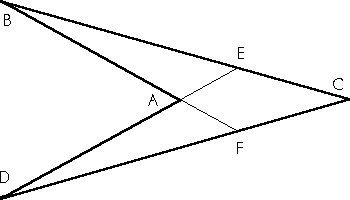Consider the kite ABCD at right. Note how triangles BCF and DCE both have the region AECF in their interiors. They thus overlap. Angle C is in common to both. Thus if we are given two additional pieces of information we may be able to prove these triangles congruent. You might consider what two pieces are required. Diagonals in regular polygons present similar situations.

The question often comes up as to how many triangles are formed by the diagonals in a polygon, perhaps regular. The answers for the triangle (1) and quadrilateral (8) border on trivial, but get rather involved for the pentagon, hexagon, etc. If you can find the value for a pentagon, this link will give you the answer for n > 5. Specifically, the sequence known as A006600 is for regular polygons and the sequence A005732 is for cyclic (inscribable in a circle) n-gons. Curiously, for n odd they are the same, whereas for n even they differ. This paper explains why.

### Tesselations

Triangulation is an important concept which we will generalize in this section. To triangulate something means either to divide into triangles or affix an object's location via triangles. Fundamentally they are based on the same concept and principles.

There are shapes other than triangles which we can use to cover a region. When using general shapes the term tesselate is used. A tesselation uses a fundamental region to completely cover (or tile) a plane such that no holes are found. This fundamental region is repeated via the various isometries (translation, rotation, reflection, glide reflection), hence they are all congruent. A regular polygon will tesselate only if the angle measure evenly divides 360°. Thus only the following regular polygons tesselate: triangles (60°), squares (90°), and hexagons (120°). Any triangle and any quadrilateral will tesselate because you can arrange them so that angles summing to 360° surround each vertex. However, only a few pentagons will tesselate and several new types were only recently discovered. Hexagons are likewise restricted.

M. C. Escher (1898-1972) was a recent Dutch artist who often utilized tesselations and other mathematical concepts, such as perspective in his works. The following link has a nice set of links to various tesselation sites and this one has a java applet useful for online study. A couple of these have contest deadlines in the March 28-April 1 range. Tesselmania demo. Tessel mania examples. Tess 1.51.

### Origami

Origami is a Japanese word referring to the art of paperfolding. Origami was developed well over 400 years ago both as a ceremonial and recreational activity. Traditionally it uses square, colored pieces of paper. Try your hand at a box, sailboat, duck, flapping bird, banger, mushroom, swan, crane, etc. Here is a site with some nice step-by-step procedures with diagrams. You will soon discover that a couple "bases", standard starting folds, are used. These include the following: waterbomb, preliminary, windmill, turtle, frog, fish, and bird bases. Remember, practice makes perfect and no one usually gets these right the first time. The directions aren't always the clearest! Following are some additional links to origami sites: 1, 2, 3, and 4. Then there are origami which tesselate: here.

### Fractals

A fractal is a geometric figure that can be separated into parts, each of which is similar to the whole. Fractals are commonly used in computer graphics to quickly dub in the leaves on trees or grass. In finance, it is the folklore which has a strong theoretical basis that market charts for stocks and currency look alike when adjusted for time and price scales. Benoit Mandelbrot helped develop the study of fractals and has a certain set named after him. We will refrain from getting more specific since complex numbers are very useful in their definition.

### Parallelograms

A parallelogram is a quadrilateral with opposite sides parallel. The following properties can now be proven. If a quadrilateral is a parallelogram, then:
1. Both pair of opposite sides are pairwise congruent;
2. Both pair of opposite angles are pairwise congruent; and
3. Diagonals intersect at their midpoints (i.e. bisect each other).
A common mistake is to assume that the diagonals are the same length, or that the diagonals bisect the angles. A quick sketch of a short, skinny and a long, skinny parallelogram should dissuade such notions. Don't forget that the distance between two parallel lines is always constant. (Proved here using parallelograms.) Parallelograms have a 2-fold rotational symmetry about the intersection of the diagonals. Not all parallelograms are reflection-symmetric, however.

In addition to the standard definition (two pairs of parallel sides), there are additional properties which are sufficient conditions to conclude that a given quadrilateral is a parallelogram. These are as follows:

1. One pair of sides is both parallel and congruent; or
2. Both pairs of opposite sides are congruent; or
3. The diagonals bisect each other; or
4. Both pairs of opposite angles are congruent.
If any one of the above conditions is met, then the quadrilateral is a parallelogram.

### Exterior Angles, Law of Sines

We will start by defining exterior angle.

 An angle is an exterior angle iff it forms a linear pair with an interior angle of a [convex] polygon.

Note: there are alternate definitions of exterior angle which may make just as much sense, but which violate the UCSMP limitation of angles being less than or equal to 180º. Please be clear which definition we are using.

Since an exterior angle of a triangle forms a linear pair with an interior angle, and that interior angle is supplementary to the sum of the other two interior angles, the following two theorems should be fairly self-evident. Please note that we refer to an exterior angle not the exterior angle since there are two possible exterior angles at each vertex.

 The measure of an exterior triangle angle is equal to the sum of the measures of the interior angles at the other two vertices (Exterior Angle Theorem). The measure of an exterior triangle angle is greater than the measure of either of the interior angles at the other two vertices (Exterior Angle Inequality).

This chapter concludes with two theorems about the lengths of sides in triangles. Specifically, the longer sides are opposite the larger angles. The text uses two theorems (Unequal sides/angles Theorems), and many nots to make this statement. This is actually a specific case of the Law of Sines given below. For nonobtuse triangles, since sine is a monotonic (always increasing or always decreasing) function between 0° and 90° it is easy to see how this works. For obtuse triangles one must also rely on the unequal angle theorem above and have deeper insight into the sin function.

 Law of Sines: In triangle ABC with sides of length a, b, and c: sin A/a = sin B/b = sin C/c     or     a/sin A = b/sin B = c/sin C.

These concepts are also often referred to as the Hinge Theorem. This basically states that given two fixed length sides in a triangle, the length of the third side will increase as the angle opposite increases, just like the set of triangles described by a hinge.

### Algebra Refresher

The chapter ends with a short algebra refresher because subsequent chapters on perimeter, area, and volume assume mastry of these essentials. The concepts reviewed here include multiplying various combinations of monomials and binomials together, factoring out a common term, finding square roots, with and without a calculator, and solving quadratics with no linear term (i.e. b = 0 or ax2 - c = 0). For further algebra background try here.

### Morley's Theorem

In 1904 Frank Morley discovered that the intersection points of adjacent angle trisectors in any triangle form an equilateral triangle.

BACK HOMEWORK ACTIVITY CONTINUE